### Home > INT2 > Chapter 10 > Lesson 10.2.5 > Problem10-108

10-108.

Solve for the variables in each of the diagrams below. Assume point $C$ is the center of the circle in part (b).

1.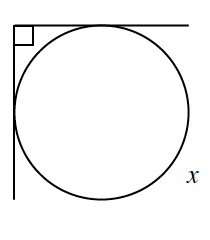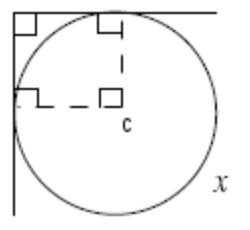$x = 360° − 90° = 270°$

1.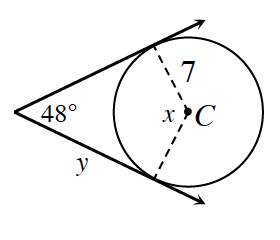$90° + 90° + 48° + x = 360°$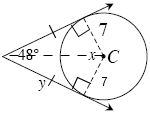By $\text{SSS} ≅$, the two triangles are congruent. Therefore, the corresponding angles of the two triangles are also congruent, which means the values of the angles are equal to $90°$, $24°$ (half the value of the original $48°$), and $66°$ (half the original value of $x$).

By the Law of Sines:

$\frac{\sin24°}{7} = \frac{\sin66°}{y}$

1.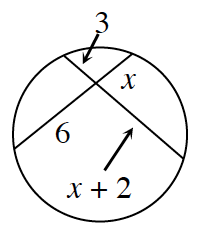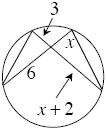The two triangles are similar, which means:

$\frac{3}{x} = \frac{6}{x + 2}$

$x = 2$